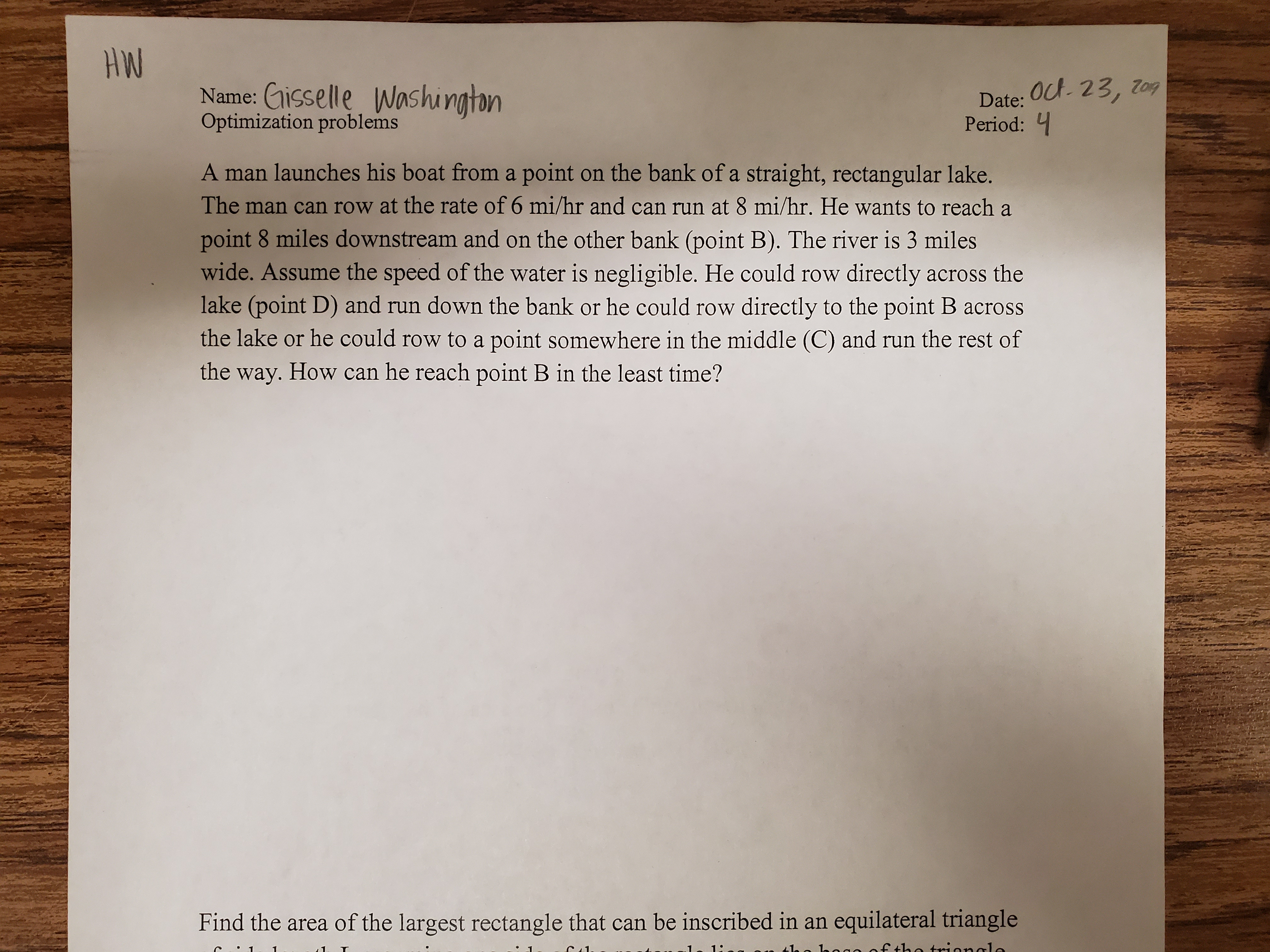# HWoct 23, 7ogName: Cisselle WashingtonOptimization problemsDate:Period:A man launches his boat froma point on the bank of a straight, rectangular lake.The man can row at the rate of 6 mi/hr and can run at 8 mi/hr. He wants to reach apoint 8 miles downstream and on the other bank (point B). The river is 3 mileswide. Assume the speed of the water is negligible. He could row directly across thelake (point D) and run down the bank or he could row directly to the point B acrossthe lake or he could row to a point somewhere in the middle (C) and run the rest ofthe way. How can he reach point B in the least time?Find the area of the largest rectangle that can be inscribed in an equilateral triangle

Question
124 viewshelp_outlineImage TranscriptioncloseHW oct 23, 7og Name: Cisselle Washington Optimization problems Date: Period: A man launches his boat froma point on the bank of a straight, rectangular lake. The man can row at the rate of 6 mi/hr and can run at 8 mi/hr. He wants to reach a point 8 miles downstream and on the other bank (point B). The river is 3 miles wide. Assume the speed of the water is negligible. He could row directly across the lake (point D) and run down the bank or he could row directly to the point B across the lake or he could row to a point somewhere in the middle (C) and run the rest of the way. How can he reach point B in the least time? Find the area of the largest rectangle that can be inscribed in an equilateral triangle fullscreen
check_circle

Step 1

Let he rows to point C which is in the middle of B and D. Then he runs to B.

Let C is x miles away from D. If x=0 then C=D , if x=8 then C=B.

So if we find x then we can find which will be the best way for him.

Step 2

CD=x, BC=8-x.

We need to find AC= ? We will use Pythagorean theorem.

AC= sqrt(x^2+9)

Step 3

Time = Distance/ Speed

For rowing Distance = sqrt(x^2+9) miles and speed= 6 mi/hr

For runnin...

### Want to see the full answer?

See Solution

#### Want to see this answer and more?

Solutions are written by subject experts who are available 24/7. Questions are typically answered within 1 hour.*

See Solution
*Response times may vary by subject and question.
Tagged in

### Calculus